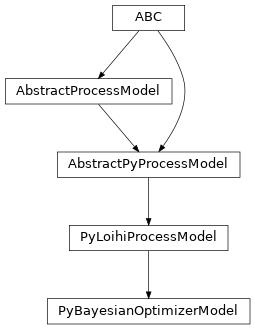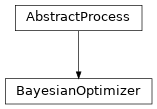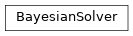# lava.lib.optimization.solvers.bayesian

## lava.lib.optimization.solvers.bayesian.modelsclass lava.lib.optimization.solvers.bayesian.models.PyBayesianOptimizerModel(proc_params=None)

__del__()

finalize the optimization processing upon runtime conclusion

Return type

`None`

acq_func_config = LavaPyType(cls=<class 'numpy.ndarray'>, d_type=<class 'numpy.ndarray'>, precision=None)
acq_opt_config = LavaPyType(cls=<class 'numpy.ndarray'>, d_type=<class 'numpy.ndarray'>, precision=None)
est_config = LavaPyType(cls=<class 'numpy.ndarray'>, d_type=<class 'numpy.ndarray'>, precision=None)
implements_process

alias of `BayesianOptimizer`

implements_protocol

alias of `LoihiProtocol`

init_search_space()

initialize the search space from the standard schema

This method is designed to convert the numpy ndarray-based search space description int scikit-optimize format compatible with all lower-level processes. Your search space should consist of three types of parameters:

1. (“continuous”, <min_value>, <max_value>, np.nan, <name>)

2. (“integer”, <min_value>, <max_value>, np.nan, <name>)

3. (“categorical”, np.nan, np.nan, <choices>, <name>)

Returns

search_space – A collection of continuous and discrete dimensions that represent the entirety of the problem search space

Return type

list[Union[Real, Integer]]

initialized = LavaPyType(cls=<class 'bool'>, d_type=<class 'bool'>, precision=None)
ip_gen_config = LavaPyType(cls=<class 'numpy.ndarray'>, d_type=<class 'numpy.ndarray'>, precision=None)
next_point_out: PyOutPort = LavaPyType(cls=<class 'lava.magma.core.model.py.ports.PyOutPortVectorDense'>, d_type=<class 'numpy.float64'>, precision=None)
num_ips = LavaPyType(cls=<class 'int'>, d_type=<class 'int'>, precision=None)
num_iterations = LavaPyType(cls=<class 'int'>, d_type=<class 'int'>, precision=None)
num_objectives = LavaPyType(cls=<class 'int'>, d_type=<class 'int'>, precision=None)
process_result_vector(vec)

parse vec into params/objectives before informing optimizer

Parameters

vec (np.ndarray) – A single array of data from the black-box process containing all parameters and objectives for a total length of num_params + num_objectives

Return type

`None`

required_resources: ty.List[ty.Type[AbstractResource]] = [<class 'lava.magma.core.resources.CPU'>]
results_in: PyInPort = LavaPyType(cls=<class 'lava.magma.core.model.py.ports.PyInPortVectorDense'>, d_type=<class 'numpy.float64'>, precision=None)
results_log = LavaPyType(cls=<class 'numpy.ndarray'>, d_type=<class 'numpy.ndarray'>, precision=None)
run_spk()

tick the model forward by one time-step

Return type

`None`

search_space = LavaPyType(cls=<class 'numpy.ndarray'>, d_type=<class 'numpy.ndarray'>, precision=None)
seed = LavaPyType(cls=<class 'int'>, d_type=<class 'int'>, precision=None)
tags: ty.List[str] = ['floating_pt']

## lava.lib.optimization.solvers.bayesian.processesclass lava.lib.optimization.solvers.bayesian.processes.BayesianOptimizer(acq_func_config, acq_opt_config, search_space, est_config, ip_gen_config, num_ips, num_objectives, seed, **kwargs)

An abstract process defining the internal state and input/output variables required by all Bayesian optimizers

## lava.lib.optimization.solvers.bayesian.solverclass lava.lib.optimization.solvers.bayesian.solver.BayesianSolver(acq_func_config, acq_opt_config, ip_gen_config, num_ips, seed, est_config={'type': 'GP'}, num_objectives=1)

Bases: `object`

The BayesianSolver is a class-based interface to abstract the details of initializing the BayesianOptimizer process and connecting it with the user’s specific black-box function

solve(name, num_iter, problem, search_space)

Conduct hyperparameter optimization for the argued problem.

Parameters
• name (str) – a unique identifier for the given experiment

• num_iter (int) – the number of Bayesian iterations to conduct

• problem (AbstractProcess) – the black-box function whose parameters are represented by the Bayesian optimizer’s search space

• search_space (np.ndarray) – At every index, your search space should consist of three types of parameters: 1) (“continuous”, <min_value>, <max_value>, np.nan, <name>) 2) (“integer”, <min_value>, <max_value>, np.nan, <name>) 3) (“categorical”, np.nan, np.nan, <choices>, <name>)

Return type

`None`

static val_init_args(acq_func_config, acq_opt_config, ip_gen_config, num_ips, seed, est_config={'type': 'GP'}, num_objectives=1)

Initialize the BayesianSolver interface.

Parameters
• acq_func_config (dict) – A dictionary to specify the function to minimize over the posterior distribution. # { # “type”: str # specify the function to minimize over the posterior # distribution: # “LCB” = lower confidence bound # “EI” = negative expected improvement # “PI” = negative probability of improvement # “gp_hedge” = probabilistically determine which of the # aforementioned functions to use at every iteration # “EIps” = negative expected improved with consideration # of the total function runtime # “PIps” = negative probability of improvement # while taking into account the total function # runtime # }

• acq_opt_config (dict) – A dictionary to specify the method to minimize the acquisition function. # { # “type” : str # specify the method to minimize the acquisition function: # “sampling” = random selection from the acquisition # function # “lbfgs” = inverse Hessian matrix estimation # “auto” = automatically configure based on the search # space # }

• ip_gen_config (dict) – A dictionary to specify the method to explore the search space before the Gaussian regressor starts to converge. # { # “type”: str # specify the method to explore the search space before the # Gaussian regressor starts to converge: # “random” = uniform distribution of random numbers # “sobol” = Sobol sequence # “halton” = Halton sequence # “hammersly” = Hammersly sequence # “lhs” = latin hypercube sequence # “grid” = uniform grid sequence # }

• num_ips (int) – The number of points to explore with the initial point generator before using the regressor.

• seed (int) – An integer seed that sets the random state increases consistency in subsequent runs.

• est_config (dict) – A dictionary to specify the type of surrogate regressor to learn the search space. #{ # “type”: str # specify the type of surrogate regressor to learn the # search space: # “GP” - gaussian process regressor #}

• num_objectives (int) – Specify the number of objectives to optimize over; currently limited to single objective.

Return type

`None`

static val_solve_args(name, num_iter, problem, search_space, num_ips, num_objectives)

validate the arguments associated with the solve method

Parameters
• name (str) – A unique identifier for the given experiment.

• num_iter (int) – The number of Bayesian iterations to conduct.

• problem (AbstractProcess) – The black-box function whose parameters are represented by the Bayesian optimizer’s search space.

• search_space (np.ndarray) – At every index, your search space should consist of three types of parameters: 1) (“continuous”, <min_value>, <max_value>, np.nan, <name>) 2) (“integer”, <min_value>, <max_value>, np.nan, <name>) 3) (“categorical”, np.nan, np.nan, <choices>, <name>)

• num_ips (int) – The number of points to explore with the initial point generator before using the regressor.

• num_objectives (int) – Specify the number of objectives to optimize over; currently limited to single objective.

Return type

`None`